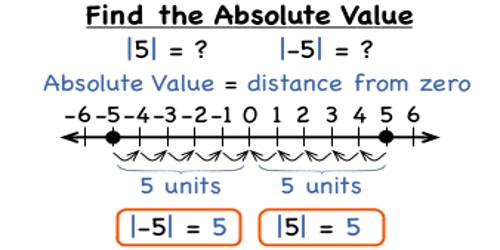Think of the distance from one integer to another as being one step. If you start at zero and take two steps to the right, you get to 2. If you start at 0 and take two steps to the left, you get to -2. If you take two steps forward, we take two steps back. We come together, ‘cuz opposites attract. In any case, we go the same distance.

Each integer (except 0) has two pieces of information: its distance from 0, and its direction from 0.

Absolute Value of a Number

The absolute value of a number is the distance of a number from zero on the number line. The absolute value is always positive since a distance is non-negative. The absolute value of an integer is the numerical value without regard to whether the sign is negative or positive. On a number line, it is the distance between the number and zero.

The absolute value of -15 is 15. The absolute value of +15 is 15

The symbol for absolute value is to enclose the number of vertical bars such as |-20| = 20 and read “The absolute value of -20 equals 20”.Examples on absolute value of an integer:

(i) Absolute value of – 7 is written as |- 7| = 7 [here mod of – 7 = 7]

(ii) Absolute value of + 2 is written as |+ 2| = 2 [here mod of + 2 = 2]

(iii) Absolute value of – 15 is written as |- 15| = 15 [here mod of – 15 = 15]

(iv) Absolute value of + 17 is written as |+ 17| = 17 [here mod of + 17 = 17]

Find the mod of:

(i) |14 – 6| = |8| = 8

(ii) – |- 10| = – 10

(iii) 15 – |- 6| = 15 – 6 = 9

(iv) 7 + |- 7| = 7 + 7 = 14

Note: Taking the negative of a number doesn’t always give us a negative number, as the previous examples demonstrate. So before assuming a number is negative just because you see a negative sign, make sure that there’s only one of them. If there are multiple negative signs, the number may be negative or positive, depending on how many negative signs there are. We know that you may not don’t never can’t want to deal with too many negative signs, but it’s just a part of life.

Information Source: